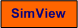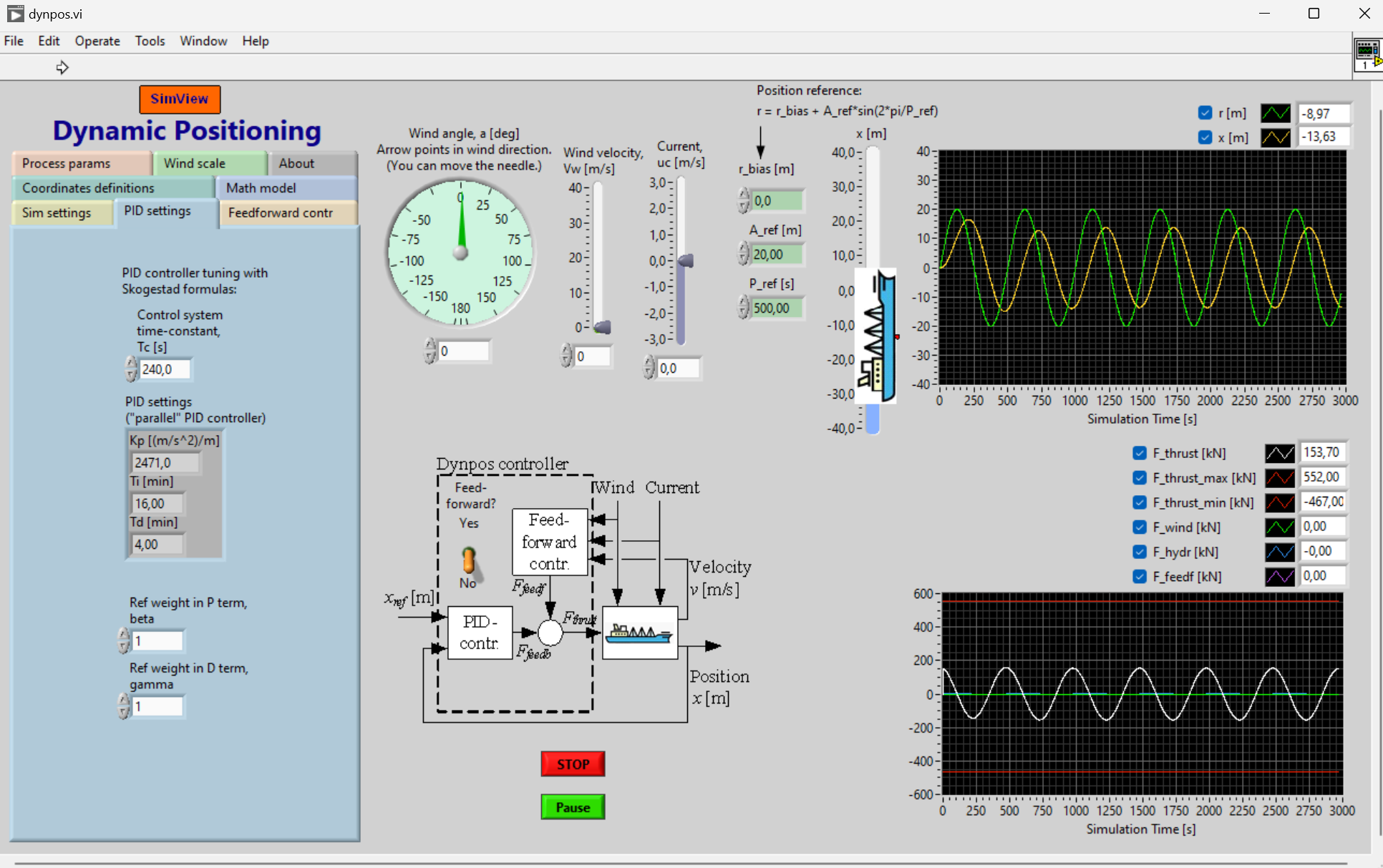# Dynamic Positioning

Snapshot of the front panel of the simulator:## Mathematical model

The mathematical model constituting the basis of the simulator is described on the tab named Math model on the simulator.

## PID tuning

PID tuning with Skogestad’s SIMC rules assuming the PID controller is on parallel form, i.e. PID = P + I + D:

Kc = 2/(Kii*Tc^2)

Ti = 4*Tc

Td = Tc

where Tc [s] is the closed loop time constant specified by the user, Kii = 1/m is the double integrator gain, and m [kg] is mass of the ship. For the controller tuning, it is assumed that the ship model is a double integrator:

m*x'' = Fp

where x [m] is the ship position, and Fp [N] is the control variable (the propeller force). This is the ship model at the critical operating point, where damping environmental forces by wind and water are neglected.

## Feedforward control

The control system includes feedforward from the position reference, and from disturbances, i.e. the environmental forces by wind and water. Feedforward control can be activated with a switch.

[SimView] [TechTeach]

Updated 31 August 2023. Developed by Finn Haugen. E-mail: finn@techteach.no.